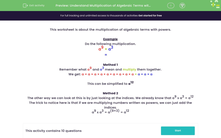# Understand Multiplication of Algebraic Terms with Powers

In this worksheet, students will multiply algebraic terms with indices or powers.Key stage:  KS 3

Curriculum topic:   Algebra

Curriculum subtopic:   Understand Expressions, Equations, Inequalities, Terms and Factors

Difficulty level:#### Worksheet Overview

This activity is about the multiplication of algebraic terms with powers.

Example

Do the following multiplication:

a9 × a3 =

Method 1

Break down what a9 and a3 mean and multiply them together.

We get: a × a × a × a × a × a × a × a × a × a × a × a

This can be simplified to a12

Method 2

The other way we can look at this is by just looking at the indices. We already know that a9 x a3 = a12

The trick to notice here is that if we are multiplying numbers written as powers, we can just add the indices.

a9 x a3 = a(9 + 3) = a12

Does that make sense? Let's test it out in some questions.

### What is EdPlace?

We're your National Curriculum aligned online education content provider helping each child succeed in English, maths and science from year 1 to GCSE. With an EdPlace account you’ll be able to track and measure progress, helping each child achieve their best. We build confidence and attainment by personalising each child’s learning at a level that suits them.

Get started••••